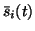Next: Initialisation of the factors Up: Model and learning algorithm Previous: Feedforward and backward computations

## Correction of the step size

In the NLFA algorithm, the step sizes of the updates of the posterior meansof the factors were corrected by taking into account the correlated effects of the updates. In NLFA the update of the posterior meansaffect the cost function through terms which result from the prior for factor si(t) and likelihood p(x(t) | s(t)). The likelihood results in several terms, one for each component of the observation vector x(t). The problem was that the update rule gives the optimal value for eachassuming that otherwise the posterior approximation stays fixed but this assumption is violated because for efficiency, all posterior means and variances are updated at once. This was remedied by utilising the Jacobian matrix of f(s(t))w.r.t. s(t).

In the NDFA algorithm the situation is slightly more complex as the prior'' p(s(t) | s(t-1)) of the factors s(t) is also affected by the factors at the previous time step, but essentially the same procedure can be used: first the effect of all the proposed updates of the posterior means of factors on x(t) and s(t) is computed, then the step is projected back to the factors. This is compared with the result which would be obtained assuming that each of the posterior means of the factors is the only parameter of the posterior approximation to change. The size of the update is then corrected by the ratio of the assumed and actual effect of the proposed updates just as in the NLFA algorithm.Next: Initialisation of the factors Up: Model and learning algorithm Previous: Feedforward and backward computations
Harri Valpola
2000-10-17